# Altitude difference

What a climb in per mille of the hill long 4 km and the altitude difference is 6 meters?

p =  1.5

### Step-by-step explanation: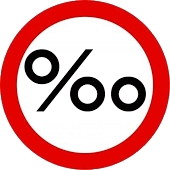Did you find an error or inaccuracy? Feel free to write us. Thank you!Tips to related online calculators
Check out our ratio calculator.
Do you want to convert length units?

#### You need to know the following knowledge to solve this word math problem:

We encourage you to watch this tutorial video on this math problem:

## Related math problems and questions:

• Climb in percentageThe height difference between points A and B is 475 m. Calculate the percentage of route climbing if the horizontal distance places A, B is 7.4 km.
• RailwaysRailways climb 7.4 ‰. Calculate the height difference between two points on the railway distant 3539 meters.
• Cablecar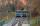Funicular on Petrin (Prague) was 408 meters long and overcomes the difference 106 meters in altitude. Calculate the angle of climb.
• ClimbFor horizontal distance 4.2 km road rise by 6.3 m. Calculate the road pitch in ‰ (permille, parts per thousand).
• Mountain railway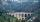Height difference between points A, B of railway line is 38.5 meters, their horizontal distance is 3.5 km. Determine average climb in permille up the track.
• Height differenceWhat height difference is overcome if we pass a road 1 km long with a pitch21 per mille?Between cities A and B is route 13 km long of stúpanie average 7‰. Calculate the height difference of cities A and B.
• Two municipalities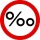The horizontal distance between municipalities is 39 km. Average sinking is 7 per mille. What is the difference in height between these municipalities?
• The railway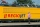The railway line has a gradient of 12 per mille. How many meters will it ascend to a horizontal distance of 4 km?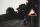A 5 km long road begins at an altitude 500 meters above sea level and ends at an altitude of 521 ASL. How many permille road rises?
• AMSL and skiingTomas skis from point A (3200m above sea level to place B. Hill has 20% descent. The horizontal distance between the start and finish is 2,5km. At what altitude is point B?
• Pedestrian up-down hill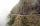Pedestrian goes for a walk first at the plane at 4 km/h, then uphill 3 km/h. Then it is in the middle of the route, turns back, and goes downhill at speed 6 km/h. The total walk was 6 hours. How many kilometers went pedestrian?
• ClimbOn the road sign, which informs the climb is 8.7%. The car drive 5 km along this road. What is the height difference that the car went to?
• What percentageWhat percentage is 12 km out of 80 km?
• In theIn the national park, the ratio of the wooded area to grassland is 4: 1. The total area is 385km2. What area is wooded?
• RailwayRailway line had on 5.8 km segment climb 9 permille. How many meters track ascent?
• Car consumption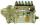The car has consumption 4.4 l/100 km. How much money will it cost ride 450 km in long if diesel price is 1.72 USD/l?